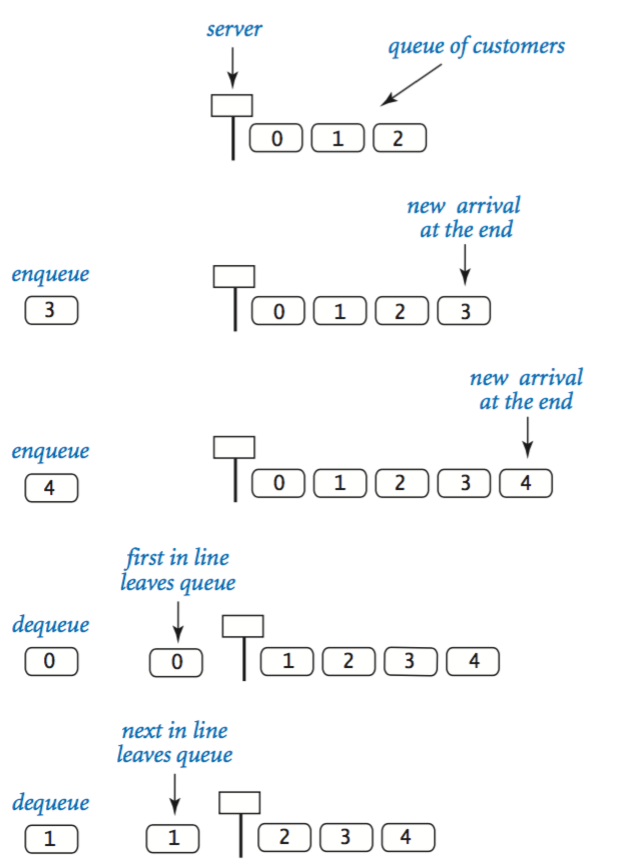Third day of Hack Reactor and we are learning the fundamentals of computer science. First up: stacks and queues!

Stacks and queues are basic ordered data structures for storing data with very specific operations. They are defined by two basic operations: inserting an item and removing an item (and by displaying what's in there, I suppose). Inserting data for stacks and queues is the same--you add it to the end. However, it is in the removal where they diverge.

### Stacks

LIFO: Last In, First Out

For me, stacks are best visualized as a stack of books.If you want one of the blue books, you'll have to remove all the books on top first before you can retrieve it. Last in, first out.

The built-in Javascript array methods that come to mind for implementing this would be .push() and .pop().

.push() inserts a value at the end of an array and .pop() removes the end value of an array.

The most basic implementation of a stack would be below:

``````var Stack = function(){
var someInstance = {};

var storage = {};
var counter = 0;

someInstance.push = function(value){
storage[counter] = value;
counter++;
};

someInstance.pop = function(){
counter--;
var value = storage[counter];
delete storage[counter];
return value;
};

return someInstance;
};
``````

Here we have rewritten push and pop, using a variable 'counter' to keep track of the number of data we currently have in our stack. This also keeps track of the last item we have in our array.

When we instantiate Stack and store the values 1 through 3...

``````var newstack = new Stack();
newstack.push(1);
newstack.push(2);
newstack.push(3);
``````

The first value we get back will be the last value we put in:

``````newstack.pop(); //--> 3
``````

### Queues

FIFO: First In, First Out

This is also quite easy to visualize: it's a line! If you have a line for ice cream, the first person in line gets ice cream first.And that's how we will retrieve information in a queue!

The two Javascript methods to implement this would be .push() and .shift().

Here's the most basic implementation of a Queue, using the method names enqueue and dequeue for push and shift.

``````var Queue = function(){
var someInstance = {};

var storage = {};
var counter = 0;
var nextUp = 0;

someInstance.enqueue = function(value){
storage[counter] = value;
counter++;
};

someInstance.dequeue = function(){
var val = storage[nextUp];
delete storage[nextUp];
nextUp++;
counter--;

return val;

};

return someInstance;
};
``````

As you can see, the biggest difference here is the use of the variable 'nextUp' and the way data is removed.

'nextUp' is used to keep track of what's at the front of the queue.

When we instantiate our Queue and store the values 1 through 3...

``````var newqueue = new Queue();
newqueue.enqueue(1);
newqueue.enqueue(2);
newqueue.enqueue(3);
``````

The first value we get back will be the first value we put in:

``````newqueue.dequeue(); //--> 1
``````

Huzzah!

Resources

These are some great resources that go in more detail:
Princeton - Stacks & Queues

Wikibooks - Stacks & Queues

CMU - Stacks & Queues

BOUN - Stacks & Queues